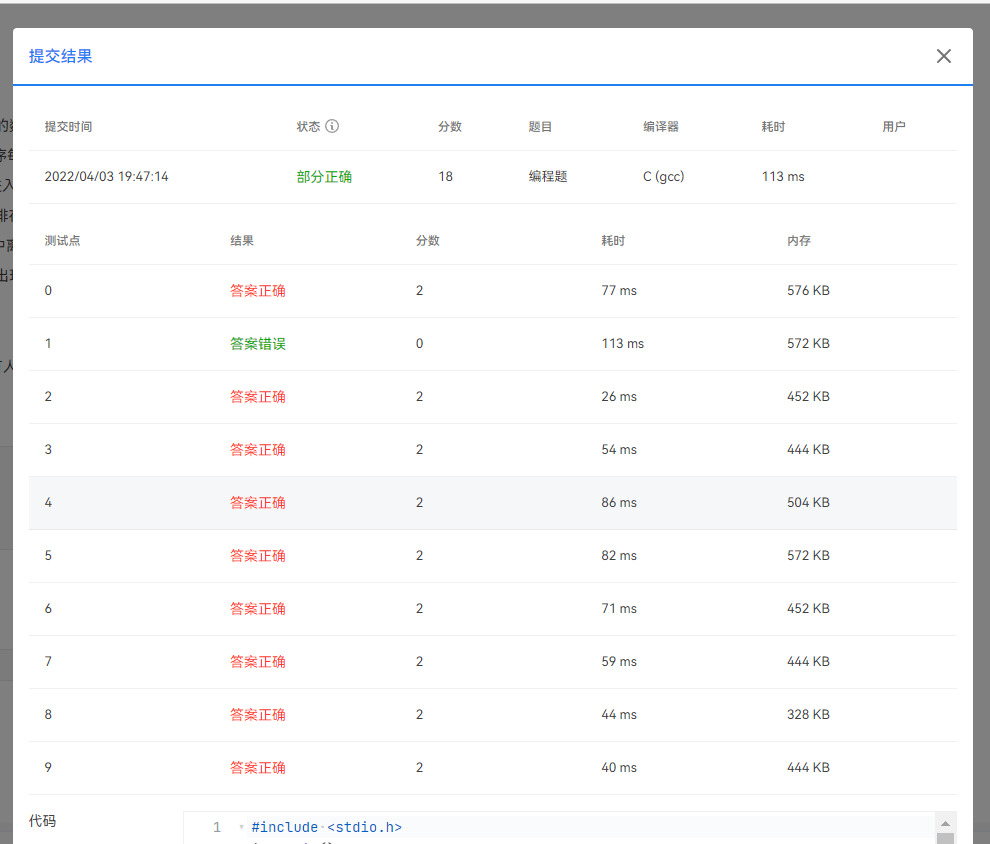# PTA 7-8 美食节 (20 分) 测试点1不正确

###### 问题遇到的现象和发生背景

I x：表示编号为x的人从队尾进入队伍

C x y:表示编号为x的人插队，排在y前一位

L x：表示编号为x的人从队伍中离开（这个人有可能还会回来）

4 4
I 1
I 2
C 3 2
C 4 1

4 1 3 2

N<=100000，M<=100000

###### 问题相关代码，请勿粘贴截图
``````#include <stdio.h>
int main()
{
int N,M,a,b,c;
int i,j,k,num=0;
char ch;
scanf("%d %d",&N,&M);
getchar();
for(i=0;i<M;i++)
{
scanf("%c",&ch);
getchar();
if(ch=='I')
{
scanf("%d",&a);
getchar();
c[num]=a;
num++;
}
else if(ch=='C')
{
scanf("%d%d",&a,&b);
getchar();
num++;
for(j=0;j<num;j++)
{
if(c[j]==b)
{
for(k=num;c[k]!=b;k--)
{
c[k]=c[k-1];
}
c[k]=a;
break;
}
}
}
else if(ch=='L')
{
scanf("%d",&a);
getchar();
num--;
for(j=0;j<num;j++)
{
if(c[j]==a)
{
for(k=j;k<num;k++)
{
c[k]=c[k+1];
}
break;
}
}
}
}
if(num==0)
printf("Bai Ren Zhan");
else
for(i=0;i<num;i++)
printf("%d ",c[i]);

}

``````
###### 运行结果及报错内容• 写回答

#### 2条回答默认 最新

•关注

num++和num--的位置不对，都应该放在for循环的下面，特别是num++那里。
代码修改如下：

``````#include <stdio.h>
int main()
{
int N,M,a,b,c;
int i,j,k,num=0;
char ch;
scanf("%d %d",&N,&M);
getchar();
for(i=0;i<M;i++)
{
scanf("%c",&ch);
getchar();
if(ch=='I')
{
scanf("%d",&a);
getchar();
c[num]=a;
num++;
}
else if(ch=='C')
{
scanf("%d%d",&a,&b);
getchar();

for(j=0;j<num;j++)
{
if(c[j]==b)
{
for(k=num;k>j;k--)
{
c[k]=c[k-1];
}
c[j]=a;
break;
}
}
num++;
}
else if(ch=='L')
{
scanf("%d",&a);
getchar();

for(j=0;j<num;j++)
{
if(c[j]==a)
{
for(k=j;k<num-1;k++)
{
c[k]=c[k+1];
}
break;
}
}
num--;
}
}
if(num==0)
printf("Bai Ren Zhan");
else
for(i=0;i<num;i++)
printf("%d ",c[i]);

}

``````
本回答被题主选为最佳回答 , 对您是否有帮助呢?
评论 编辑记录

#### 悬赏问题

• ¥15 VB6.0中PICTUREBOX加载本地图片无法显示
• ¥100 关于游戏app session获取的问题
• ¥15 MYSQL数据库建表
• ¥15 爬虫程序爬取TTGChina网站文章代码
• ¥35 由于系统缓冲区空间不足或队列已满，不能执行套接字上的操作。
• ¥15 如何用下图方法在AMESim中搭建离心泵模型
• ¥15 C#连接服务器，请求时报Ssl/Tsl未能建立安全通道
• ¥15 xcode15build的c++ dylib在10.15上不兼容
• ¥15 CPLD如何实现在线逻辑分析
• ¥15 控制面板卸载无权限！！